Author: Oscar Cronquist Article last updated on April 18, 2018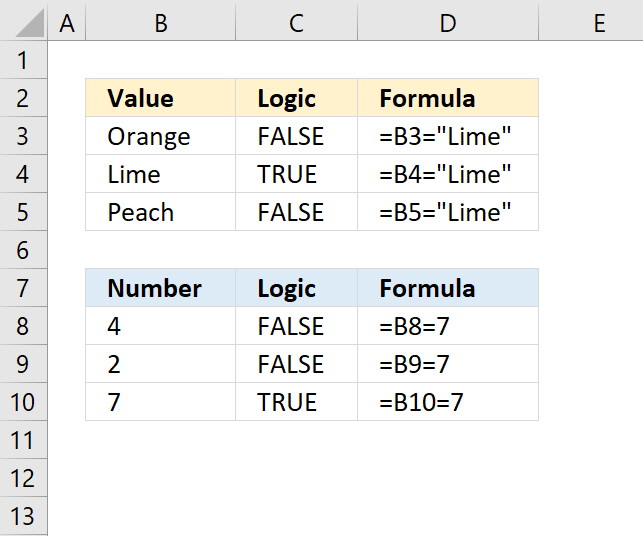The easiest way to check if a cell has a value is, in my opinion, to use the equal sign to compare the cell value with the value you are looking for.

The equal sign is a logical operator that turns the formula a logical expression and returns a boolean value, TRUE or FALSE.

Formula in cell C3:

=B3="Lime"

Use double quotation marks before and after the text value in order to do the comparison. B3 is a cell reference to a specific cell at the intersection of column B and row 3.

Copy cell C3 and paste to cells below and the cell reference changes in each cell. B3 is, therefore, a relative cell reference.

If you forget the double quotation marks Excel will evaluate your text value as a built-in function or a user-defined function. If such function can't be found Excel returns #NAME? error.

Formula in cell D8:

=B8=7

Don't use double quotation marks with numbers, if you do Excel will evaluate your number as a text value and the comparison will never return TRUE.

Example,

=B10="7"

returns FALSE even though cell B10 has number 7.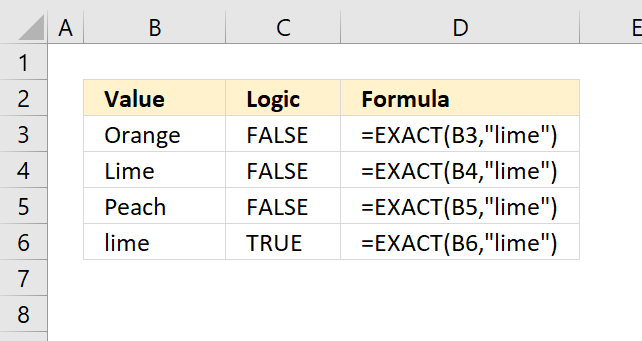The comparison made by the equal sign is, however, not case-sensitive. Use the EXACT function to perform a case-sensitive comparison, see picture above.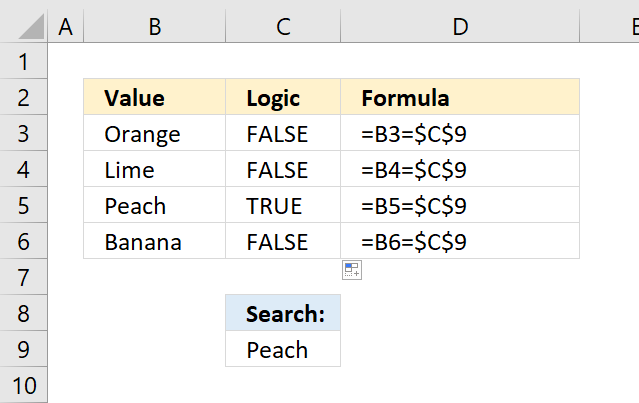A formula like this one has one of the values hardcoded into the formula:

=B3="Lime"

If you want to compare another value you must change each formula in every cell which is not at all efficient.

The picture above shows a formula that compares two cell values, however, one of the cell references are locked to cell C9.

How did I enter this formula?

1. Double press with left mouse button on cell C3 with left mouse button.
2. Type =B3=C9
3. Press function key F4 to automatically change C9 to \$C\$9.
4. Press Enter
5. Copy cell C3
6. Paste to cells below.

If you now examine the formula you will see that the first cell reference B3 in the formula changes in each cell.

This happens with relative cell reference whereas an absolute cell reference doesn't change.

Having cell references in your formulas allows you to quickly change a value without adjusting the cell formulas at all.

Try it yourself, change the value in cell C9 to Banana and check column C for changes. Cell C6 is now TRUE and C5 is FALSE.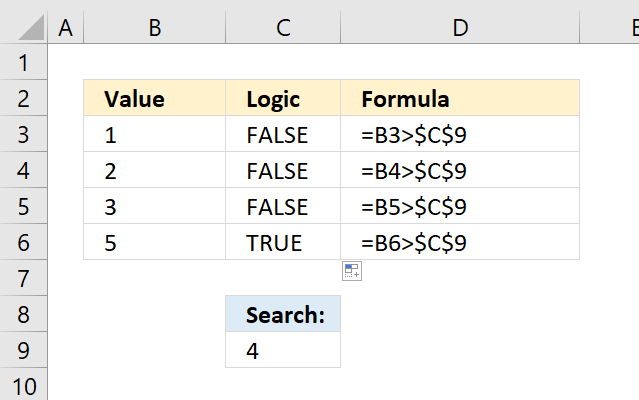The equal sign (=) is one out of 3 different logical operators, the picture above shows you the greater than sign (>).

You can combine these three logical operators to perform different logical expressions, see table below.

 Logical operator Description Example < less than B3<\$C\$9 > greater than B3>\$C\$9 <= less than or equal to B3<=\$C\$9 >= greater than or equal to B3>=\$C\$9 = equal to B3=\$C\$9 <> not equal to B3<>\$C\$9

The formula in column C returns TRUE if the value in column B is larger than 4.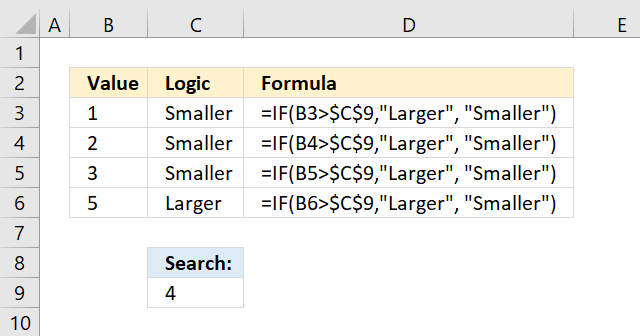Now you know what a logical expression is which is an essential part of an IF function.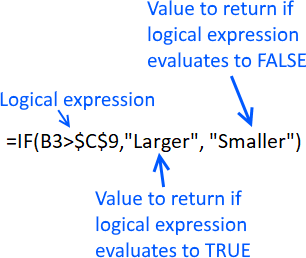The IF function has three arguments and the first one uses a logical expression to determine which out of two values to return.

### Get Excel *.xlsx file

If cell has value.xlsx